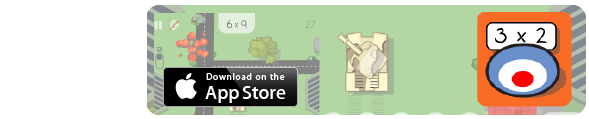## Fraction Finder

Fraction Finder is a mobile-friendly fraction demonstration tool.

Y2:To write simple fractions, for example half of 6 = 3 and recognise the equivalence of 1/2 and 2/2.
Y3:To recognise, find and write fractions of a discrete set of objects: unit fractions and non-unit fractions with small denominators

This resource models a successful pen and paper strategy for finding fractions of a number.

Step One: Press 'Next', (keep pressing until you get a fraction you'd like to model)
Step Two: Read the problem, e.g. 1/2 of 10
Step Three: Read the sentence
Step Four: Change the white denominator to match the problem
Step Five: Change the yellow numerator to match the problem
Step Six: Share the dots equally between the boxes
Step Seven: Count the dots in the yellow boxes to find the answer.

Made using theandlibraries.